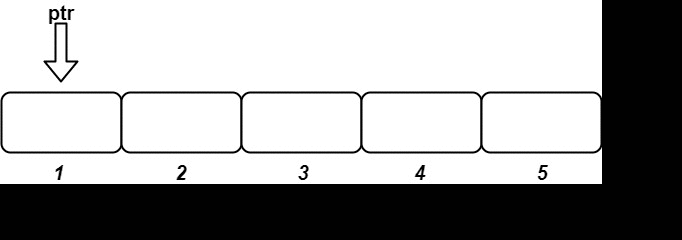# 1656. Design an Ordered Stream | LeetCode Solution

## 1656. Design an Ordered Stream

There is a stream of `n` `(idKey, value)` pairs arriving in an arbitrary order, where `idKey` is an integer between `1` and `n` and `value` is a string. No two pairs have the same `id`.

Design a stream that returns the values in increasing order of their IDs by returning a chunk (list) of values after each insertion. The concatenation of all the chunks should result in a list of the sorted values.

Implement the `OrderedStream` class:

• `OrderedStream(int n)` Constructs the stream to take `n` values.
• `String[] insert(int idKey, String value)` Inserts the pair `(idKey, value)` into the stream, then returns the largest possible chunk of currently inserted values that appear next in the order.

Example:```Input
["OrderedStream", "insert", "insert", "insert", "insert", "insert"]
[, [3, "ccccc"], [1, "aaaaa"], [2, "bbbbb"], [5, "eeeee"], [4, "ddddd"]]```
```Output
[null, [], ["aaaaa"], ["bbbbb", "ccccc"], [], ["ddddd", "eeeee"]]```
```Explanation
// Note that the values ordered by ID is ["aaaaa", "bbbbb", "ccccc", "ddddd", "eeeee"].
OrderedStream os = new OrderedStream(5);
os.insert(3, "ccccc"); // Inserts (3, "ccccc"), returns [].
os.insert(1, "aaaaa"); // Inserts (1, "aaaaa"), returns ["aaaaa"].
os.insert(2, "bbbbb"); // Inserts (2, "bbbbb"), returns ["bbbbb", "ccccc"].
os.insert(5, "eeeee"); // Inserts (5, "eeeee"), returns [].
os.insert(4, "ddddd"); // Inserts (4, "ddddd"), returns ["ddddd", "eeeee"].
// Concatentating all the chunks returned:
// [] + ["aaaaa"] + ["bbbbb", "ccccc"] + [] + ["ddddd", "eeeee"] = ["aaaaa", "bbbbb", "ccccc", "ddddd", "eeeee"]
// The resulting order is the same as the order above.
```

Constraints:

• `1 <= n <= 1000`
• `1 <= id <= n`
• `value.length == 5`
• `value` consists only of lowercase letters.
• Each call to `insert` will have a unique `id.`
• Exactly `n` calls will be made to `insert`.

### Solution:

The explanation given is a bit tricky and not easy to understand. After reading the problem statement 3-4 times you will start getting what the question is saying.
Basically, in every insert function, we will get one index and a string corresponding to that index. This is easy to maintain via an array or map, then what's the problem here?? The problem is returning a vector of strings so that after concatenating all the vectors we get the real sequence.

Suppose we get the values in the insert function in the ascending order from 1 to n. So in each insert function, we will simply return the same string we are getting.

But if we are getting the numbers in random order then we need to maintain order. Until we don't get one we will return an empty vector. If we get one we will return the set of strings from index 1 to x such that every number is present between x.